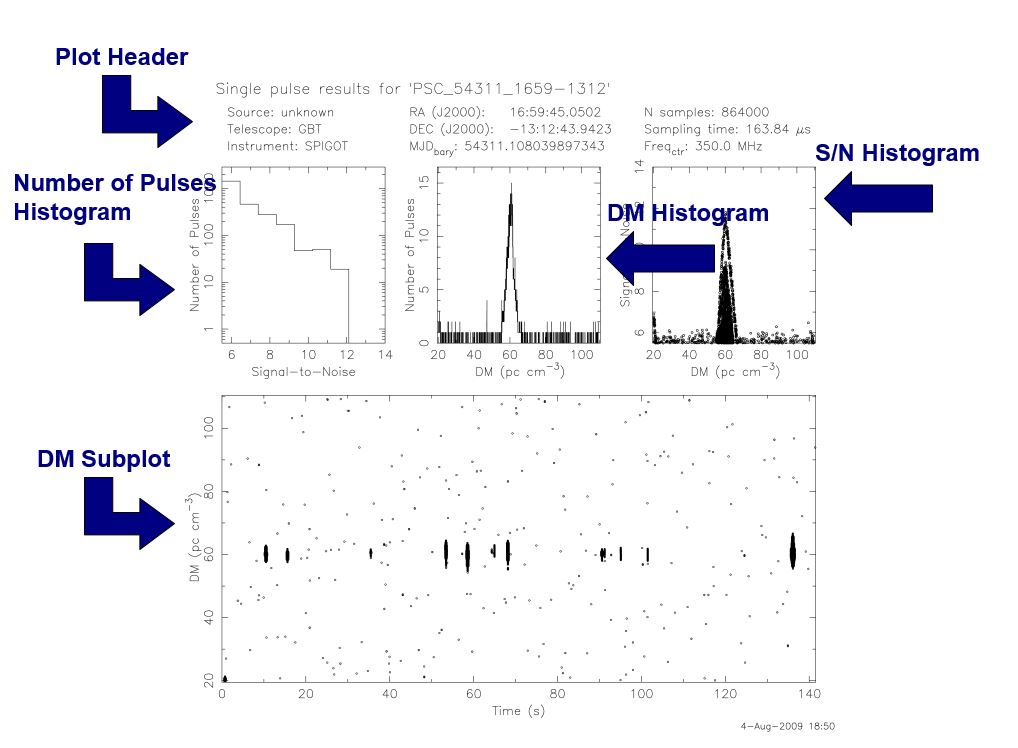## Knowledge Base

### Practice Plots

When you score pulsar data plots you will be asked to score each sub-plot separately. If enough of the subplots look like a pulsar then it’s worth conducting a follow-up observation with the GBT!

In Prepfold Plots, the subplots you will grade are: Integrated Pulse Profile, Time Domain Plot, Sub-band Plot and DM Plot.In Single Pulse Plots you will grade the DM subplot, S/N Histogram, and DM Histogram.First Look at the plot below.  What grade would you give each of the subplots?  Your choices for each subplot are are “not a pulsar”, “maybe a pulsar” and “pulsar”.

There are several plots in each embedded slide show below. Each time you click on the image, a grade and an explanation will appear for a subplot.  Try scoring these plots yourself, or together as a class or club.  Advance to a new plot at the bottom of the slide deck using the right arrow. Discuss on the forum!

Prep-fold Periodicity Plots:

Single Pulse Plots:

Last updated